Time: 3 Hours                                                                                                     Max. Marks: 100

NOTE: There are 9 Questions in all.

·      Question 1 is compulsory and carries 20 marks. Answer to Q. 1. must be written in the space provided for it in the answer book supplied and nowhere else.

·      Out of the remaining EIGHT Questions answer any FIVE Questions. Each question carries 16 marks.

·      Any required data not explicitly given, may be suitably assumed and stated.

Q.1       Choose the correct or best alternative in the following:                                         (2x10)

a.       The image of the line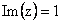under the mapping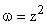is a

(A)  Circle.                                          (B)  Parabola.

(C)    Hyperbola.                                  (D)  Ellipse.

b.      The values of z for which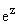is real is

(A)    any multiple of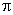.                         (B)  odd multiples of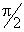.

(C)  all multiples of.                       (D)  any real number.

c.   The value of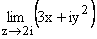where z = x i y is

(A)    2                                                  (B)  0

(C)  - 4i                                               (D)  i

d.   Let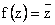.  Then which of the following is true:

(A)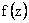is not continuous at z = 0

(B)is differentiable at z = 0

(C)is not continuous at z = 0 but differentiable at z = 0

(D)is continuous at z = 0 but not differentiable at z = 0

e.   The value of integral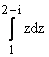is

(A)     0                                                  (B)  1

(C)  1+2i                                             (D)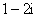f.    The angle between the surfaces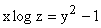and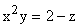at the point (1, 1, 1) is

(A)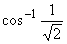(B)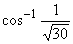(C)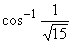(D)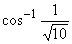g.   A force field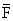is said to be conservative if

(A)     Curl= 0                                   (B)  grad= 0

(C)  Div= 0                                    (D)  Curl (grad) = 0

h.   The partial differential equation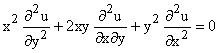is an example of

(A)    Hyperbolic equation                     (B) Elliptic equation

(C) Parabolic equation                         (D) None of these

i.    The inequality between mean and variance of Binomial distribution which is true is

(A)   Mean < Variance                          (B) Mean = Variance

(C) Mean > Variance                          (D) Mean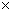Variance = 1

j.    Let f (x) be a probability density function defined by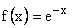, for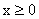and f (x) = 0 for x < 0, then the value of cumulative distribution function at x =2 is

(A)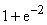(B)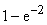(C)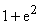(D)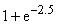Answer any FIVE Questions out of EIGHT Questions.

Each question carries 16 marks.

Q.2     a.   Show that the function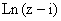is analytic everywhere except on the half line y =1 , x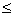0.                                                                       (8)

b.   If u is a harmonic function of two variables (x,y),  then show that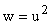is not a harmonic function, unless u is a constant.                                        (8)

Q.3     a.   Evaluate the integral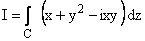from point 1-2i to point 2-i along the curve C,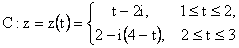.                                                             (8)

b.   Find the residue of the function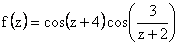at z =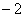.             (8)

Q.4     a.   Find all possible Taylor’s and Laurent series expansions of the function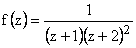about the point z = 1.                                          (10)

b.   Evaluate the integral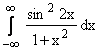, using contour integration.                          (6)

Q.5     a.   Find the directional derivative of the scalar point function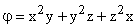at the point (2, 2, 2) in the direction of the normal to the surface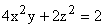at the point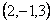.      (6)

b.   If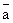and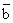are constant vectors and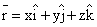show that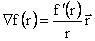and hence show that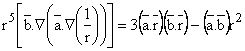, where r =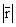(10)

Q.6     a.   Find the value of the surface integral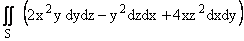where S is the curved surface of the cylinder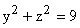bounded by the planes x = 0, x = 2.                                        (8)

b.   The vector field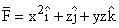is defined over the volume of the cuboid given by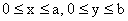,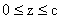. Evaluate the surface integral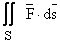, where S is the surface of the cuboid.     (8)

Q.7     a.   A tightly stretched string with end points fixed at x = 0 and x = L, is initially at rest in equilibrium state.  If it is set vibrating by giving to each of its points a velocity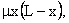find the displacement of the string at any point x from one end, at any point of time t.                                                      (12)

b.   Evaluate the integral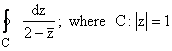, traversed anti-clockwise.       (4)

Q.8     a.   A continuous type random variable X has probability density f(x) which is proportional to x2 and X takes values in the interval [0, 2]. Find the distribution function of the random variable use this to find P (X >1.2) and conditional probability P(X > 1.2/ X>1).                                                      (8)

b.   A firm plans to bid Rs.300 per tonne for a contract to supply 1000 tonnes of a metal.  It has two competitors A and B and it assumes that the probability that A will bid less than 300/- per tonne is 0.3 and that B will bid less than Rs.300 per tonne is 0.7.  If the lowest bidder gets all the business and the firms bid independently, what is the expected value of business in rupees to the firm.                                                (8)

Q.9     a.   Suppose that on an average 1 house in 1000 houses gets fire in a year in a district.  If there are 2000 houses in that district find the probability that exactly 5 houses will have fire during the year. Also find approximate probability using Poisson distribution.   (8)

b.   Derive the mean and variance of binomial distribution.                                         (8)